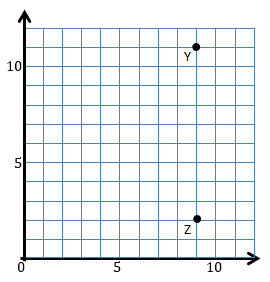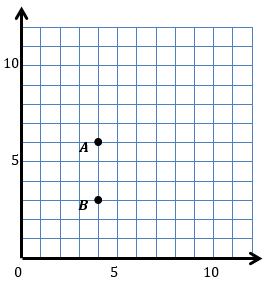# Patterns and the Coordinate Plane

## Objective

Plot horizontal and vertical lines on a coordinate plane and find patterns in their coordinates.

## Common Core Standards

### Core Standards

?

• 5.G.A.1 — Use a pair of perpendicular number lines, called axes, to define a coordinate system, with the intersection of the lines (the origin) arranged to coincide with the 0 on each line and a given point in the plane located by using an ordered pair of numbers, called its coordinates. Understand that the first number indicates how far to travel from the origin in the direction of one axis, and the second number indicates how far to travel in the direction of the second axis, with the convention that the names of the two axes and the coordinates correspond (e.g., x-axis and x-coordinate, y-axis and y-coordinate).

• 5.G.A.2 — Represent real world and mathematical problems by graphing points in the first quadrant of the coordinate plane, and interpret coordinate values of points in the context of the situation.

## Criteria for Success

?

1. Draw horizontal and vertical lines in the coordinate plane.
2. Understand that horizontal lines are parallel to the x-axis and perpendicular to the y-axis and vertical lines are perpendicular to the x-axis and parallel to the y-axis.
3. Discern the pattern that horizontal lines all have the same y-value and vertical lines all have the same x-value.
4. Name the coordinates of a point that lies on a horizontal or vertical line, including without needing or being able to plot that point.

## Tips for Teachers

?

• The following material is needed for today's lesson: grid paper
• You can print copies of this Inch Grid Paper if you do not have grid paper already.

#### Fishtank Plus

• Problem Set
• Student Handout Editor
• Vocabulary Package

?

### Problem 1

Amiyah and Brian were playing Battleship. Brian guessed the coordinate pair (5, 8) and got a hit. What coordinates would you recommend Brian give for his next guess? Explain your reasoning.

### Problem 2

Draw a line that passes through points Y and Z.1. Plot one other point on the same line as $\overleftrightarrow{YZ}$ and label it X.
2. List the coordinates of XY, and Z in the following table.
 Point Coordinates X Y Z
1. What do you notice about the coordinates of points XY, and Z? What do you wonder?

#### References

Grade 5, Unit 9, Lesson 3 (2017-2018) is made available by Achievement First as a part of their Open Source web portal under a CC BY 4.0 license. Copyright © 1999-2017 Achievement First. Accessed May 25, 2018, 3:30 p.m..

Modified by The Match Foundation, Inc.

### Problem 3

Use the table below to answer the following questions.

 Point Coordinates H (4, 2.5) I (10, 2.5) J (8, 2.5)
1. Before plotting the points, what do you notice about their coordinates? What do you wonder?
2. Plot the points on a coordinate grid. Then connect the points by drawing a line through all of them.
3. Determine the coordinates of another point that would lie on this line.

#### References

EngageNY Mathematics Grade 5 Mathematics > Module 6 > Topic A > Lesson 5Concept Development

Grade 5 Mathematics > Module 6 > Topic A > Lesson 5 of the New York State Common Core Mathematics Curriculum from EngageNY and Great Minds. © 2015 Great Minds. Licensed by EngageNY of the New York State Education Department under the CC BY-NC-SA 3.0 US license. Accessed Dec. 2, 2016, 5:15 p.m..

Modified by The Match Foundation, Inc.

## Problem Set & Homework

#### Discussion of Problem Set

• In #1, what’s the relationship of line e to the x-axis and y-axis?
• What do all of the points of line e have in common in #1f?
• In #2, how did you know that the points given in parts (a) and (c) were on a line that was not parallel to the x-axis? For the lines that were parallel to the x-axis, what was the distance of every point on those lines from the x-axis?
• In #3, how did you know that the points given in parts (a) and (b) were on a line that was not parallel to the y-axis?
• In #4, what’s the relationship of line h to the x-axis and y-axis?
• What pattern occurs in the coordinate pairs that let you know that line h is vertical in #4d?

?

1. Use a straightedge to construct a line that goes through points A and B.  Label the line l.
2. Which axis is parallel to line l?Which axis is perpendicular to line l?

1. Plot two more points on line l.  Name them C and D.
2. Give the coordinates of each point below.

A:  ___________ B:  ___________

C:  ___________ D:  ___________

1. Give the coordinates of another point that falls on line l that isn’t shown in the coordinate plane drawn above.

#### References

EngageNY Mathematics Grade 5 Mathematics > Module 6 > Topic A > Lesson 5Exit Ticket

Grade 5 Mathematics > Module 6 > Topic A > Lesson 5 of the New York State Common Core Mathematics Curriculum from EngageNY and Great Minds. © 2015 Great Minds. Licensed by EngageNY of the New York State Education Department under the CC BY-NC-SA 3.0 US license. Accessed Dec. 2, 2016, 5:15 p.m..

Modified by The Match Foundation, Inc.

### Mastery Response

?